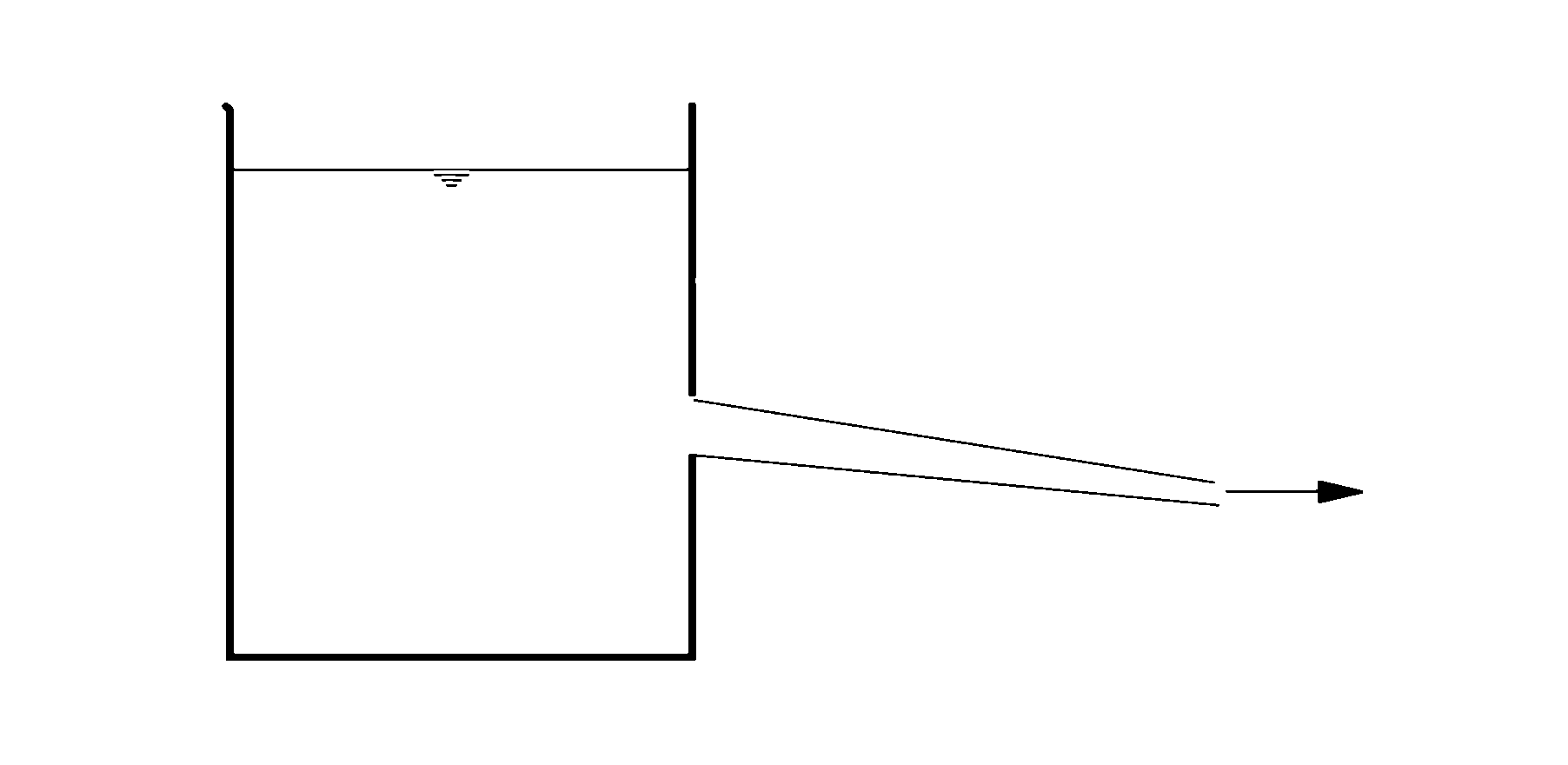# 第3章 流体运动学--课后作业【含答案】 水力学

（1）多选题

A.流体质点的一般运动规律为 $x=(a+1)e^t-t-1$, $y=(b+1)e^t-t-1$

B.无法求出流体质点的一般运动规律

C.拉格朗日变数下流体质点的加速度为 $a_x=(a+1)e^t$, $a_y=(a+1)e^t$

D.无法求得欧拉变数下流体质点的加速度

A  A

B  B

C  C

D  D

（2）单选题

A  2

B  -10

C  0

D  -58

（3）单选题

A  对

B  错

（4）单选题

A  对

B  错

（5）单选题A  恒定均匀流

B  非恒定均匀流

C  恒定非均匀流

D  非恒定非均匀流

（6）多选题

A  流动为平面二维均匀流动

B  流线为圆心在原点的同心圆

C  迹线为圆心在原点的同心圆

D  流动为有旋流动

（7）单选题

A  对

B  错

（8）多选题

A.断面平均流速$v=\frac{1}{2}u_{max}$

B.断面平均流速 $v=\frac{7}{8}u_{max}$

C.流量 $Q=\frac{6}{7}u_{max}bh$

D.流量 $Q=\frac{7}{8}u_{max}bh$

A  A

B  B

C  C

D  D

（9）单选题

A  管径大的地方过流流量大

B  管径大的地方过流流量小

C  管径大的地方平均流速小

D  管径大的地方平均流速大